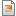## Action Items Plugin

Due date Description State
2017-02-10 Write Master thersis edit

## Latex Plugin

This is an example of the %BEGINLATEX{inline="1" density="200" gamma="1.0" color="Purple"}%\LaTeX%ENDLATEX% rendering possibilities using the LatexModePlugin.

The singular value decomposition of a matrix is defined as %BEGINLATEX{label="one" color="Green"}% \begin{displaymath} A = U \Sigma V^H \end{displaymath} %ENDLATEX% where and are both matrices with orthonormal columns, indicates a complex-conjugate transpose, and is a diagonal matrix with singular values along the main diagonal. Eq. %REFLATEX{one}% is just one of the many matrix decompositions that exists for matrix .

## Math Plugin

The following will only display correctly if this plugin is installed and configured correctly.

\int_{-\infty}^\infty e^{-\alpha x^2} dx = \sqrt{\frac{\pi}{\alpha}}

\int_{-\infty}^\infty e^{-\alpha x^2} dx = \sqrt{\frac{\pi}{\alpha}}

{\cal P} & = & \{f_1, f_2, \ldots, f_m\} \ {\cal C} & = & \{c_1, c_2, \ldots, c_m\} \ {\cal N} & = & \{n_1, n_2, \ldots, n_m\}

{\cal P} & = & \{f_1, f_2, \ldots, f_m\} \ {\cal C} & = & \{c_1, c_2, \ldots, c_m\} \ {\cal N} & = & \{n_1, n_2, \ldots, n_m\}

\cal A, B, C, D, E, F, G, H, I, J, K, L, M, \ \cal N, O, P, Q, R, S, T, U, V, W, X, Y, Z

\cal A, B, C, D, E, F, G, H, I, J, K, L, M, \ \cal N, O, P, Q, R, S, T, U, V, W, X, Y, Z

\sum_{i_1, i_2, \ldots, i_n} \pi * i + \sigma

\sum_{i_1, i_2, \ldots, i_n} \pi * i + \sigma

--

Topic attachments
I Attachment History Action Size Date Who Commentjpg DSC_0783.JPG r1 manage 3168.9 K 2016-04-01 - 05:28 KojiNakamurajpg DSC_1033.JPG r1 manage 4139.9 K 2016-04-01 - 05:27 KojiNakamurajpg DSC_1209.JPG r1 manage 3897.5 K 2016-04-01 - 05:27 KojiNakamura
Topic revision: r4 - 2017-09-25 - KojiNakamuraCopyright © 2008-2023 by the contributing authors. All material on this collaboration platform is the property of the contributing authors.
Ideas, requests, problems regarding TWiki? Send feedback